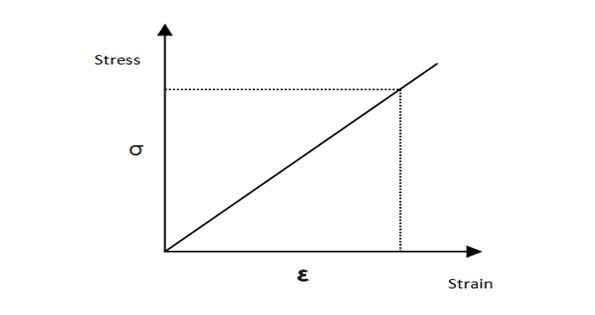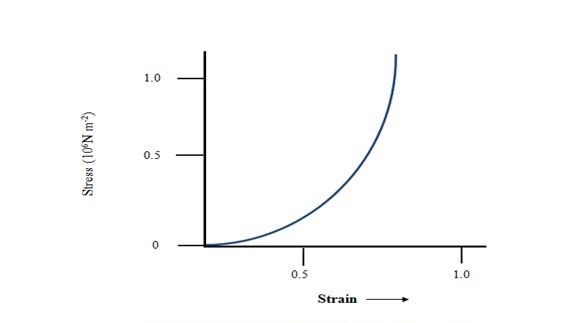# Stress

## Introduction

The application of a deforming force can make an object deform. Further, in the process of getting back the object into the actual shape, another force is required. From inside the object, an opposing force gets released. Different types of stress can be categorised in different formats based on the action performed. Moreover, stress is applied to deform the body. A curve line can represent the relation between the stress-strain visually. In this tutorial, detailed information about stress has been described with types and Hooke’s law.

## Information Regarding Stress

A solid item has a fixed shape and size followed from the time of construction. In order to change the original size and shape or deform the body, force is required. The amount of force applied or required to deform an object in the unit per area is the stress. Stress is symmetrical to strain (Scool. co, 2022). We can put it as an equation,

Stress = force / cross-sectional area.

Moreover, Strain is represented as an attachment to the length in each unit.

Therefore, the amount of applied force on an area can be symmetrical to the area of the unit while measuring it. When forces have involved in an object in such a method that the object is unmoving and remains in the fixed equilibrium, it is deformed to a little or enormous extent relying upon the character of the material of the object and the importance of the deforming power. The deformation may not be detectable visually in numerous materials.

When an object is presented through a force which is deforming, a stimulating force is produced in the object also. This restoring strength is equal in importance but works in the opposite direction to the involved force. The restoring strength per unit area is comprehended as stress. Hence, the Magnitude of the stress = F/A## Types of Stress

Several classes of stress in physics are available, however, it is primarily categorised into two conditions that are Normal Stress and Tangential or Shearing Stress. Different stress types are concerned with the following attributes:

### Normal Stress

Normal stress is generally applied to an object when the principle of the deforming force is vertical to the cross-sectional area of the targeted object. The measurement of the wire or the magnitude of the object modifies the amount of applied stress at normal. Normal stress can be additionally organised into two classifications based on the measurement of applied strength on the unit per area.

### Longitudinal Stress

The situation, where the deforming stresses are acting like the length of the object is known as the longitudinal stress. It can also stretch or compress the object with its length. The result of this stress application lead to the consequences affects changes in the diameter of the object.

### Volumetric stress

At the stage of application of the deforming stress, the applied force results in changing the volume from the dimension. In the volume stress, the object’s volume gets changes due to the application of the deforming forces.

### Tensile stress

The application of the deforming force can make result in an increment in the length of the object, where the stress is being applied. Moreover, an opposite or equal force is applied by stretching from both ends resulting in the Tensile stress.

### Compressive Stress

In the opposite situation where the application of force is resulting in a decreasing the amount of the length of the object, where a force is being applied is known as the Compressive stress. Additionally, the Tensile or compressive stress can furthermore be phrased as longitudinal stress.The connection between the strain and stress for a delivered object underneath tensile stress can be discovered experimentally. In a common test of the belongings of tensile, an involved force pulls a wire. The fractional difference in length (the strain) and the force that is applied is required to generate the strain are documented. The involved force is slowly expanded in stages and the difference in measurement is recorded. A diagram can be drawn with the curved line between the stress which is comparable in importance to the devoted force per unit area and the produced strain to comprehend the process. Additionally, the curved line in between the comprehension of the relation between the strain and stress can be varied based on the object itself.

## Hooke’s Law

The stain and stress take distinctive formations in different situations. When the deformation amount of stress is small, the strain and stresses are symmetrical to each other. This relationship between stress and strain needs to be fixed (Onheaven.co, 2022). Additionally, Hooke’s law is adequate in terms of strain and stress and it's also justified for almost every object.

Images Coming soon

## Conclusion

In this tutorial, the deformation pressure on an object is highly successfully, along with the relationship between strain and stress. The stress-strain demeanour contrasts from object to object. More frequently, the objects that are facing the strength are applying force for deforming is stated as the application of stress. The proportional relation between the strain and stress can be comprehended easily with the application of the involved force. This can be formed from the tutorial that the strain occurs to be a unit less amount.

## FAQs

Q.1. What stress is actually?

Ans. The measurement of the force that is relying on the per unit area of an object to make an alternation of the object’s shape is stress. Moreover, stress is an external force which is useful to make deformation of an object.

Q.2. What is the connection between the stress-strain?

Ans. The connection between the two properties, strain and stress are symmetrical to each other with the elastic limit. This relationship has been explained in Hooke’s law.

Q.3. What is the concept of stress and strain that is applied in different areas?

Ans. The notion of stress is applied in other engineering techniques. The concept is reliable and required to be comprehended in similar fields to gain knowledge about the external forces that can affect certainly.

Updated on: 09-Jan-2023

122 Views